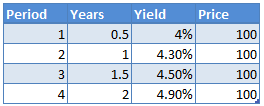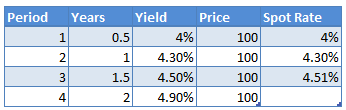# Bootstrapping Spot Rate Curve (Zero Curve)

A spot rate curve, also known as a zero curve refers to the yield curve constructed using the spot rates such as Treasury spot rates instead of the yields.

A spot rate Treasury curve is more suitable to price bonds because most bonds provide multiple cash flows (coupons) to the bond holders at different points in time, and it is better to use the spot rates as the discount rates for different time periods rather than using a single discount rate.

We can build a spot rate curve using on-the-run treasuries, off-the-run treasuries, or both. In this article we will learn how to construct build a spot rate curve using a method called bootstrapping. In the bootstrapping technique one repetitively applies a no-arbitrage implied forward rate equation to yields on the estimated Treasury par yield curve. Given below is the step-by-step process to arrive at the spot curve using the bootstrapping method.

Step 1: Decide on the Instrument for Yield Curve

The spot curve can be obtained by using on-the-run Treasury securities, off-the-run treasury securities, or a combination of both, or Treasury coupon strips. The instrument you select should not have credit risk, liquidity risk, embedded options, or any pricing anomalies. In our example, let’s use on-the-run Treasury yields.

Step 2: Select the Par Yield Curve

Typically, you will not find Treasury securities for only a few maturities such as 3-month, 6-month, 2-year, 5-year, 10-year, and 30 years.

Step 3: Interpolate the Missing Yields

We will have to interpolate the in between yields for different periods, such as for 3-year, 3-year, 6-year, and so on. This can be done using the simple interpolation formula however it will not be very accurate.

Step 4: Calculate Spot Rates Using Treasury Yields

In this step we will apply the bootstrapping method to calculate the spot rates.

To reiterate, the spot curve is made up of spot interest rates for zero coupon bonds of different maturities. For example, a 2-year spot rate tells us for the interest rate is for a zero-coupon bond of two-year maturity. We bootstrap this data from the Treasury yield curve.

Let’s say we have the following data:Let’s use the above data to calculate the spot rates. Note that first two securities, i.e., the 6 month and 1 year Treasury securities are T-bills which are discount securities, essentially zero-coupon securities. So, for these the spot rate will be the same as the yield, i.e., 4% and 4.3%.

0.5 year spot rate, z1 = 4%

1 year spot rate, z2 = 4.3%

We can now use this data to calculate the 1.5 year spot rate. Since 1.5 year bond is selling at par, its coupon will be 4.5%. The three cash flows are:

Cash flow at 0.5 year = 100*0.045*0.5 = 2.25

Cash flow at 1 year = 100*0.045*0.5 = 2.25

Cash flow at 1.5 year = 100 + 100*0.045*0.5 = 102.25

We want the 1.5 year zero rate such that the present value of all cash flows discounted by their respective spot rates is equal to the bond’s price i.e., 100.

100 = 2.25/(1+4%/2)^1 + 2.25/(1+4.3%/2)^2 + 102.25/(1+z3/2)^3

Solving for z3, we get z3 = 4.511, which is the 1.5 year spot rate.When we plot the spot rates against the maturities, we get the spot rate or the zero curve.